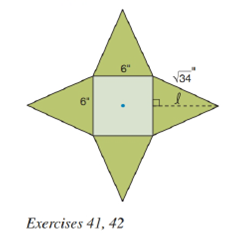Chapter 9.2, Problem 42EElementary Geometry For College St...

7th Edition
Alexander + 2 others
ISBN: 9781337614085

Solutions

Chapter
SectionElementary Geometry For College St...

7th Edition
Alexander + 2 others
ISBN: 9781337614085
Textbook Problem

Find the volume of the regular square pyramid that was formed in Exercise 41.To determine

To find:

The volume of the regular square pyramid.

Explanation

Given:

The four congruent isosceles triangles are folded upward it forms a regular square pyramid and the figure given below

From question 41, the slant height is 5" and base area is B=36in2.

Properties Used:

The volume V of a pyramid having a base area B and an altitude of length h is given by V=13Bh.

According to the Pythagorean theorem, in a right-angled triangle

hypotenuse2=base2+perpendicular2.

The area of the square is side2.

Approach Used:

i) Calculate the apothem of a square base a=side2.

ii) Using the property l2=a2+h2, find the height or altitude h by substituting the values of l and a.

iii) Calculate the base area B of the square base formed.

iv) Substitute the values of area B and the height h in equation V=13Bh to find the value of volume V.

Calculation:

From the figure below,

considering one of the lateral faces which is an isosceles triangle

Still sussing out bartleby?

Check out a sample textbook solution.

See a sample solution

The Solution to Your Study Problems

Bartleby provides explanations to thousands of textbook problems written by our experts, many with advanced degrees!

Get Started

Find more solutions based on key concepts